# Title, subtitle, caption and tag in ggplot2

When using ggplot2 you can set a title, a subtitle, a caption and a tag. There are two ways to add titles: using `ggtitle` or `labs` function. The former is only for titles and subtitles and the latter also allows adding tags and captions.

## Title

Option 1. Using `ggtitle`

``````# install.packages(ggplot2)
library(ggplot2)

ggplot(economics, aes(date, unemploy)) +
geom_area(fill = rgb(0, 0.5, 1, alpha = 0.5)) +
ggtitle("Title of the plot using ggtitle()")``````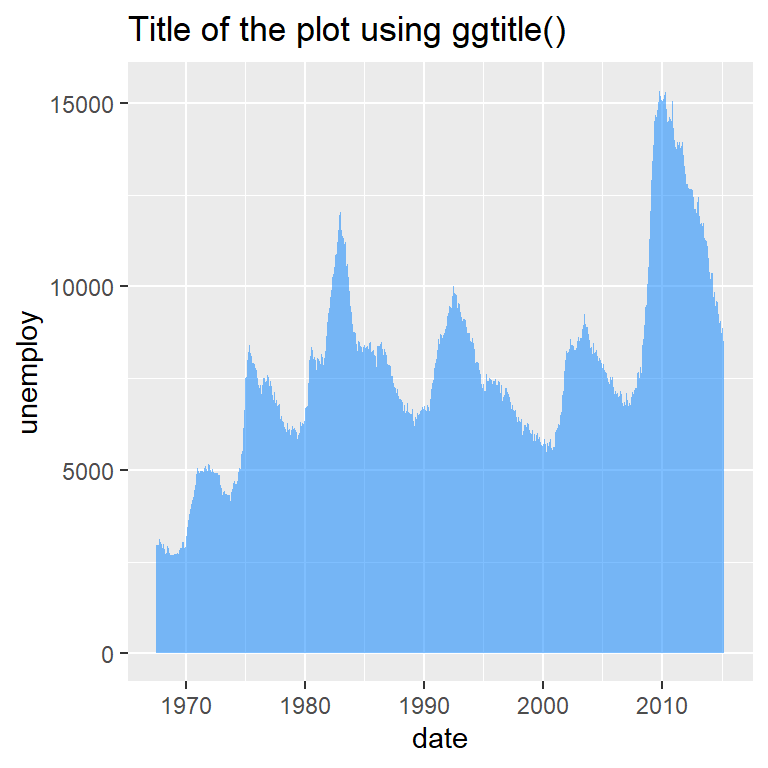Option 2. Using `labs`

You can also use the `labs` function to add the title.

``````# install.packages(ggplot2)
library(ggplot2)

ggplot(economics, aes(date, unemploy)) +
geom_area(fill = rgb(0, 0.5, 1, alpha = 0.5)) +
labs(title = "Title of the plot using labs()")``````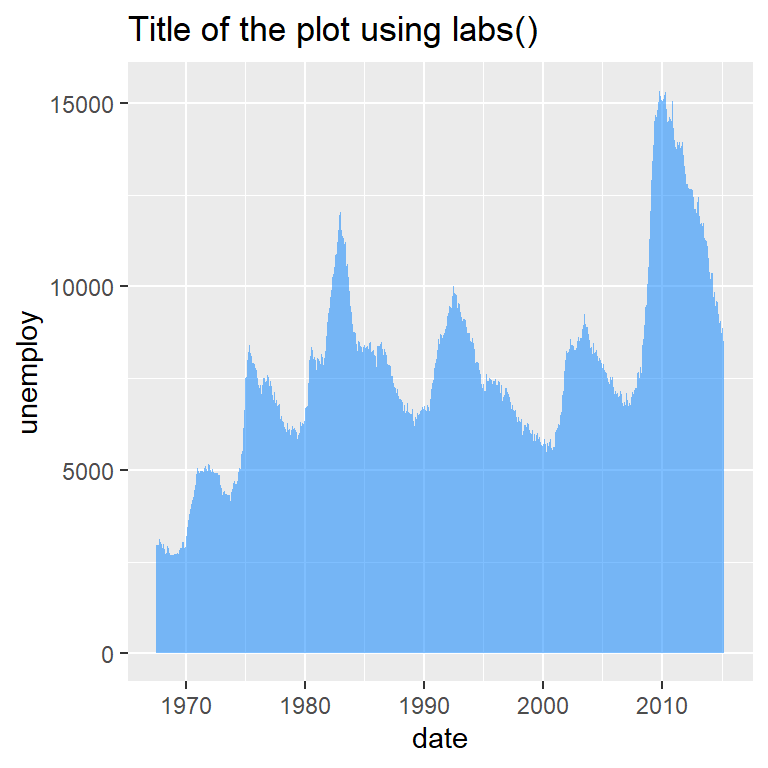Title position

The title position can be set respect to the whole plot instead of the panel with the `plot.title.position` component of the `theme` function. Default value is `"panel"`. This configuration also applies to the subtitle.

``````# install.packages(ggplot2)
library(ggplot2)

ggplot(economics, aes(date, unemploy)) +
geom_area(fill = rgb(0, 0.5, 1, alpha = 0.5)) +
labs(title = "Title on the plot margin") +
theme(plot.title.position = "plot")``````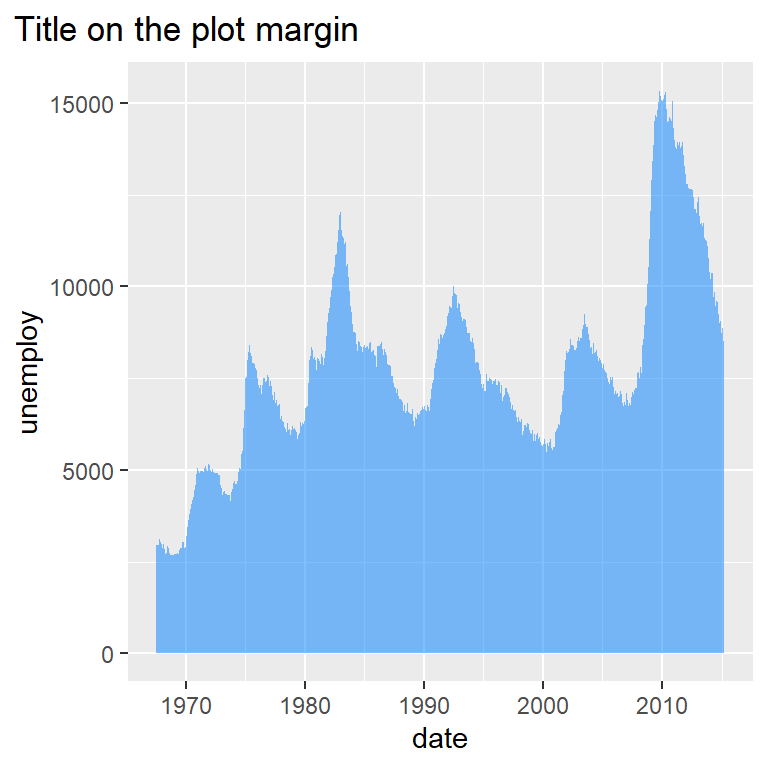## Subtitle

You can add a subtitle the same way you added the title, but with the `subtitle` argument.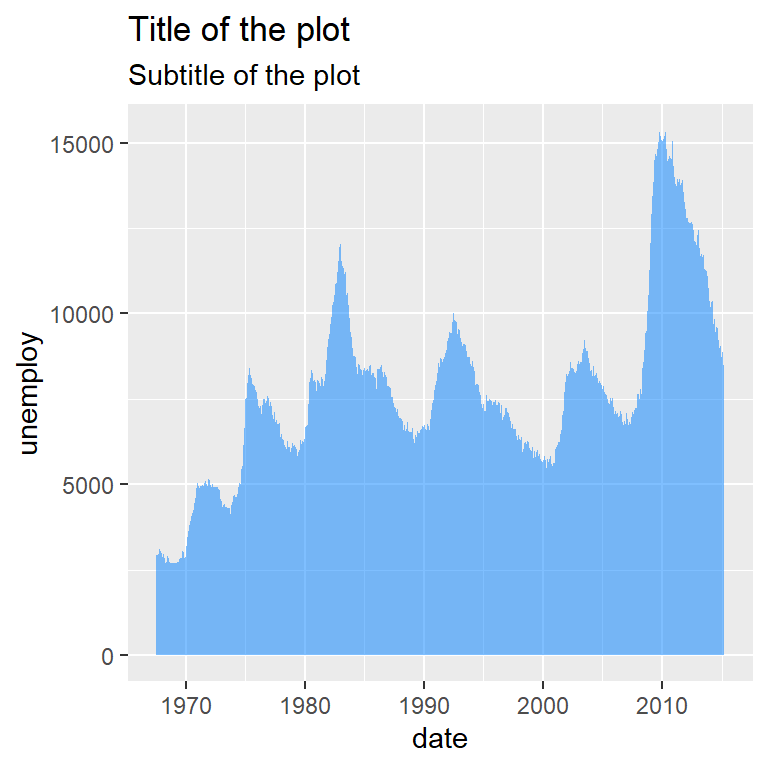Option 1. Using `ggtitle`

``````# install.packages(ggplot2)
library(ggplot2)

ggplot(economics, aes(date, unemploy)) +
geom_area(fill = rgb(0, 0.5, 1, alpha = 0.5)) +
ggtitle("Title of the plot",
subtitle = "Subtitle of the plot")``````Option 2. Using `labs`

``````# install.packages(ggplot2)
library(ggplot2)

ggplot(economics, aes(date, unemploy)) +
geom_area(fill = rgb(0, 0.5, 1, alpha = 0.5)) +
labs(title = "Title of the plot",
subtitle = "Subtitle of the plot")``````

## Caption

A caption can be used to describe the figure. You can add it with the `caption` argument of the `labs` function.

``````# install.packages(ggplot2)
library(ggplot2)

ggplot(economics, aes(date, unemploy)) +
geom_area(fill = rgb(0, 0.5, 1, alpha = 0.5)) +
labs(title = "Title of the plot",
subtitle = "Subtitle of the plot",
caption = "This is the caption")``````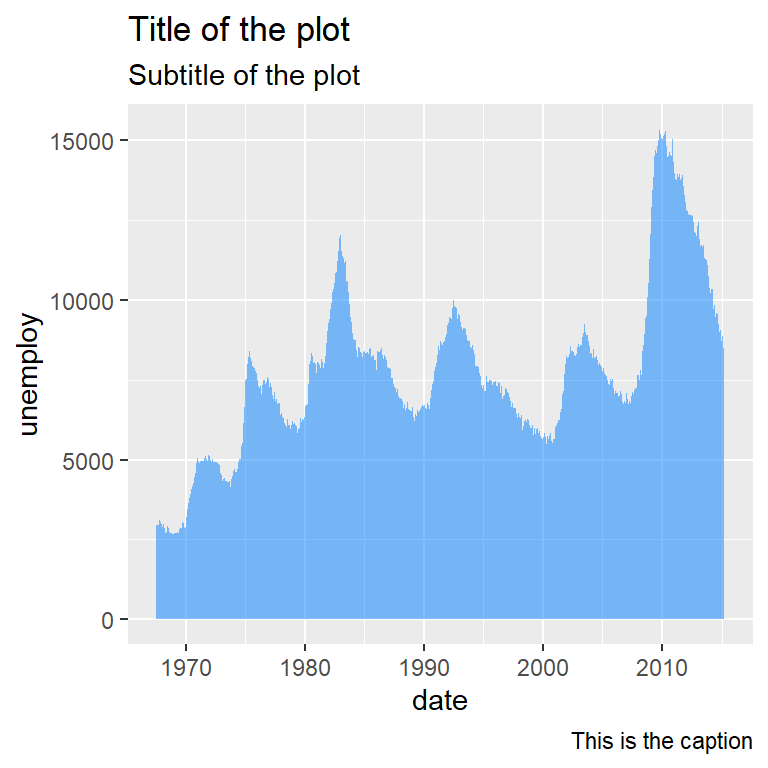The `plot.caption.position` of the `theme` function allows aliging the caption to the panel (`"panel"`, default) or the whole plot (“`plot`”).

``````# install.packages(ggplot2)
library(ggplot2)

ggplot(economics, aes(date, unemploy)) +
geom_area(fill = rgb(0, 0.5, 1, alpha = 0.5)) +
labs(caption = "This is the caption") +
theme(plot.caption.position = "plot",
plot.caption = element_text(hjust = 0))``````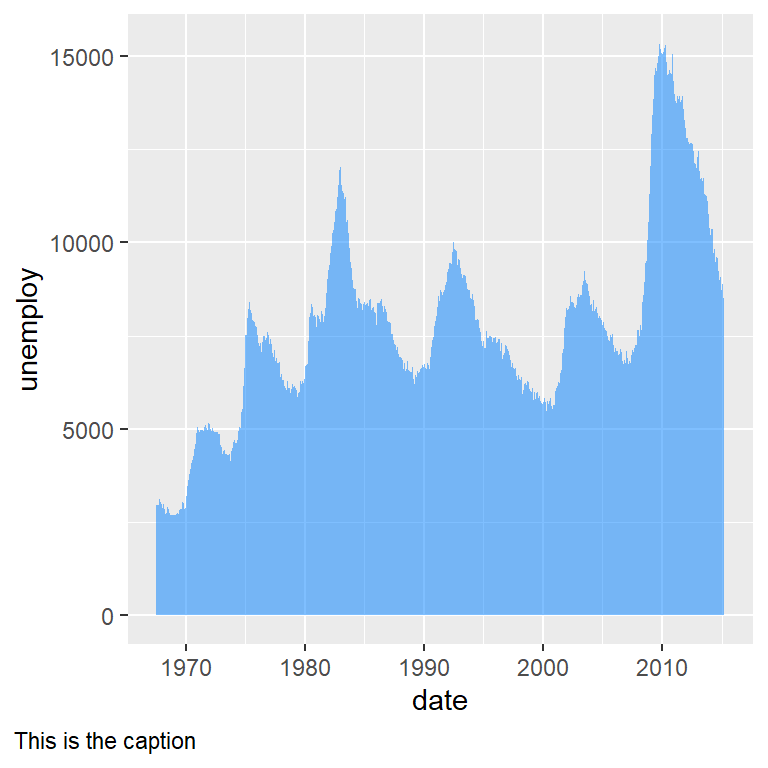Note that the default for the caption is right alignment, so you can set `hjust = 0` to move the caption to the left of the whole plot.

## Tag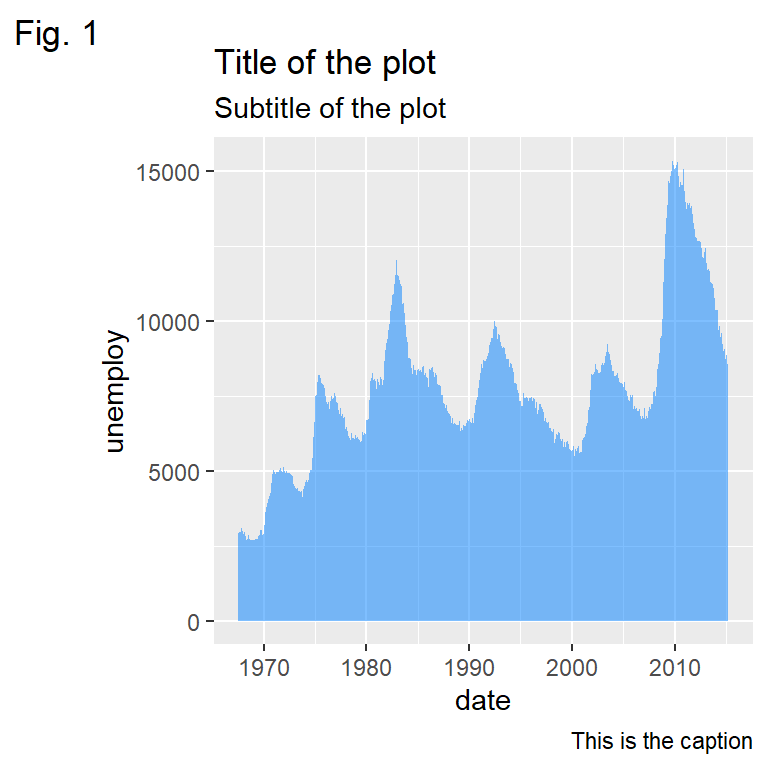Tags are useful to indicate the figure numbering. You can add it with the `tag` argument of the `labs` function.

``````# install.packages(ggplot2)
library(ggplot2)

ggplot(economics, aes(date, unemploy)) +
geom_area(fill = rgb(0, 0.5, 1, alpha = 0.5)) +
labs(title = "Title of the plot",
subtitle = "Subtitle of the plot",
caption = "This is the caption",
tag = "Fig. 1")``````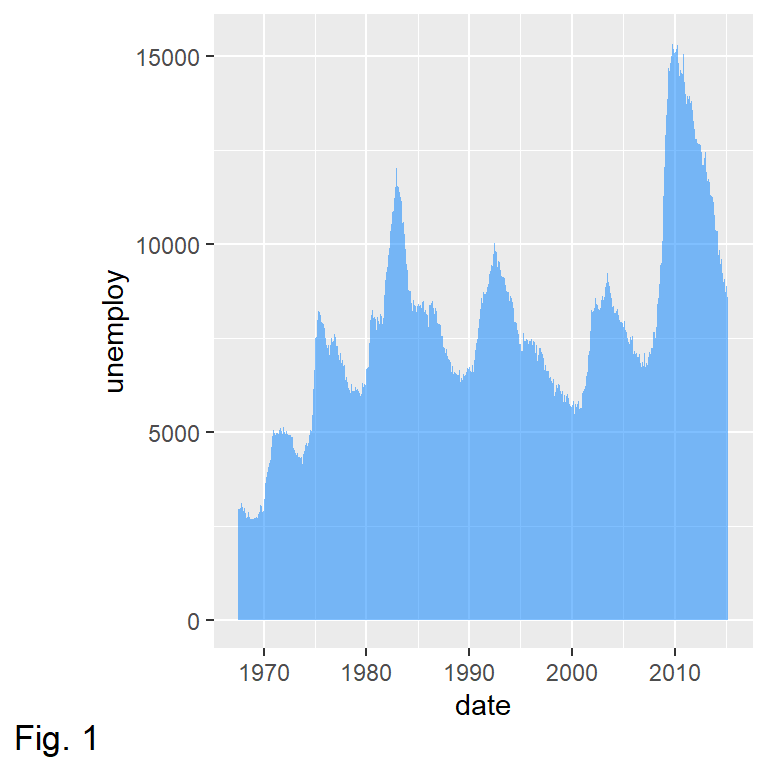Tag position

The position of the tag can be set with the `plot.tag.position` component of the `theme` function. Possible values are `"topleft"` (default), "`top"`, `"topright"`, `"left"`, `"right"`, `"bottomleft"`, `"bottom"` or `"bottomright"`.

``````# install.packages(ggplot2)
library(ggplot2)

ggplot(economics, aes(date, unemploy)) +
geom_area(fill = rgb(0, 0.5, 1, alpha = 0.5)) +
labs(tag = "Fig. 1") +
theme(plot.tag.position = "bottomright)``````

The titles, subtitles, captions and tags can be customized with the `plot.title`, `plot.subtitle`, `plot.caption` and `plot.tag` components of the `theme` function, making use of `element_text`. You can modify the color, the font family, the text size, the text face, the angle or the vertical and horizontal adjustment for each text as in the example below.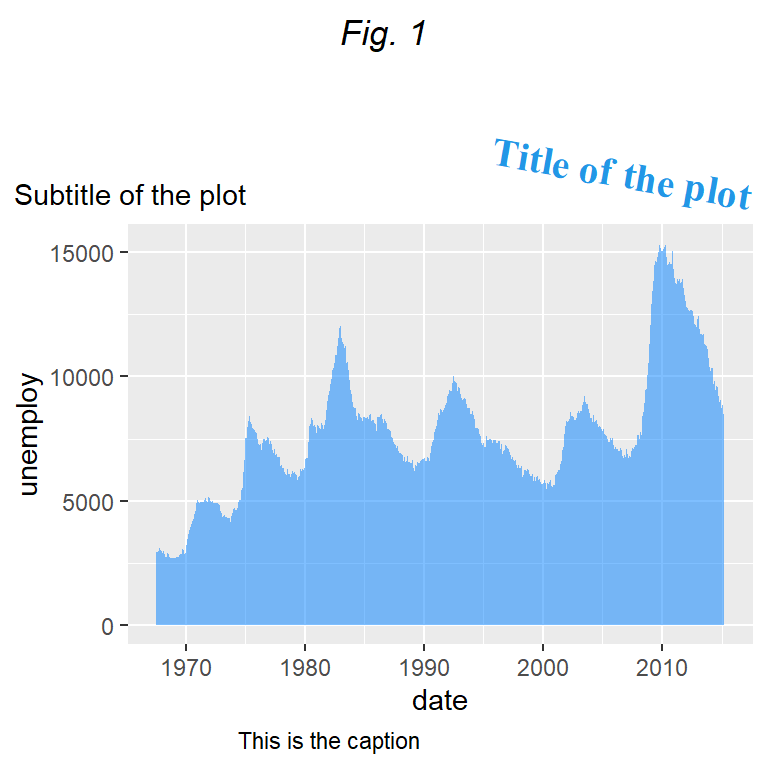``````# install.packages(ggplot2)
library(ggplot2)

ggplot(economics, aes(date, unemploy)) +
geom_area(fill = rgb(0, 0.5, 1, alpha = 0.5)) +
labs(title = "Title of the plot",
subtitle = "Subtitle of the plot",
caption = "This is the caption",
tag = "Fig. 1") +
theme(plot.title = element_text(family = "serif",              # Font family
face = "bold",                 # Font face
color = 4,                     # Font color
size = 15,                     # Font size
hjust = 1,                     # Horizontal adjustment
vjust = 1,                     # Vertical adjustment
angle = -10,                   # Font angle
lineheight = 1,                # Line spacing
margin = margin(20, 0, 0, 0)), # Margins (t, r, b, l)
plot.subtitle = element_text(hjust = 0),    # Subtitle customization
plot.caption = element_text(hjust = 0.25),  # Caption customization
plot.tag = element_text(face = "italic"),   # Tag customization
plot.title.position = "plot",               # Title and subtitle position ("plot" or "panel")
plot.caption.position = "panel",            # Caption position ("plot" or "panel")
plot.tag.position = "top")                  # Tag position``````